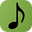#### Pythagorean Tuning

Attributed to Pythagoras (ca. 569 BC - ca. 475 BC), it is the first documented tuning system.

Pythagoras calculated the mathematical ratios of intervals using an instrument called the monochord. He divided a string into two equal parts and then compared the sound produced by the half part with the sound produced by the whole string. An octave interval was produced: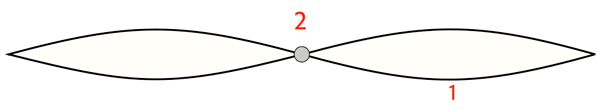Thus concludes that the octave mathematical ratio is 2 to 1.

By dividing the string into 3 equal parts and vibrating 2 of these parts we obtain the fifth interval: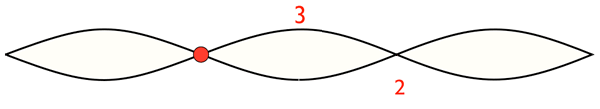Thus concludes that the fifth mathematical ratio is 3 to 2.

By dividing the string into 4 equal parts and vibrating 3 of these parts we obtain the fourth interval:Thus concludes that the fourth mathematical ratio is 4 to 3.

Using this method Pythagoras calculates the same ratios we calculated using the harmonics series.

##### Scale Construction

From a C, we will build a major scale according to the Pythagorean tuning. We first calculate the fifth by multiplying the frequency of C by 3/2 (fifth size):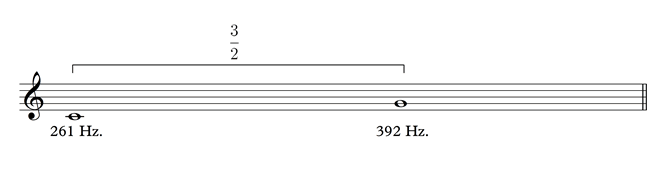To multiply a number by a fraction we multiply by the numerator (top number) and then divide by the denominator (bottom number).
G = 261 x 3 / 2.

From G we calculate D by dividing the frequency of G by 4/3 (quarter size):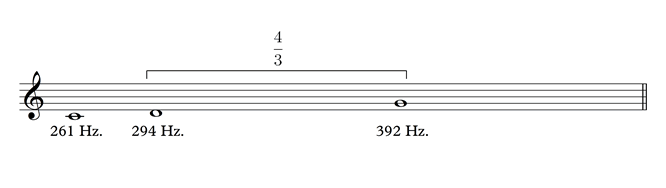To divide a number by a fraction we multiply by the denominator (bottom number) and then divide by the numerator (top number).
Re = 392 x 3 / 4.

Now A (D x 3/2):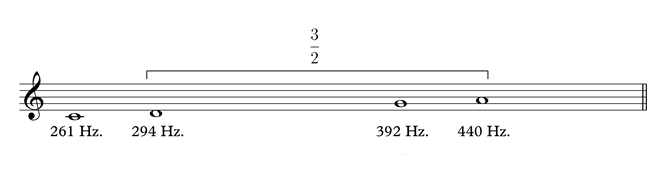Then E (A divided by 4/3):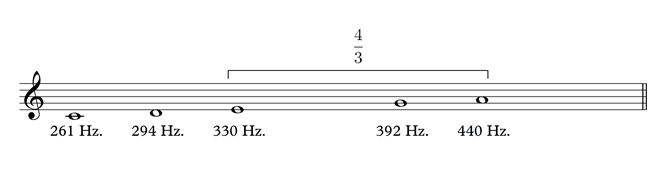Now B (E x 3/2):F equals C x 3/2 and C5 is C4 x 2: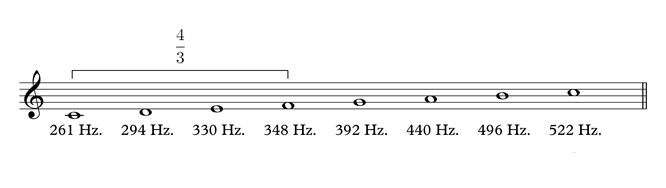Pythagorean tuning remains in use until the Renaissance, what leads us to seek a new tuning system?

The mathematical ratio of the major third in the harmonic series is 5/4 or 1.25, what is the ratio of the third in the Pythagorean scale? Dividing the frequency of E (330) by C (261) we realize that this third is larger than the third in the harmonic series:

330 ÷ 261 = 1.2643678161

Pythagorean tuning was effective when the commonly used harmonic intervals were the octave, fifth and fourth. With the increasing use of thirds during the Middle Ages and the Renaissance, the Pythagorean third was no longer acceptable to many ears. This leads us to seek new tuning systems ...